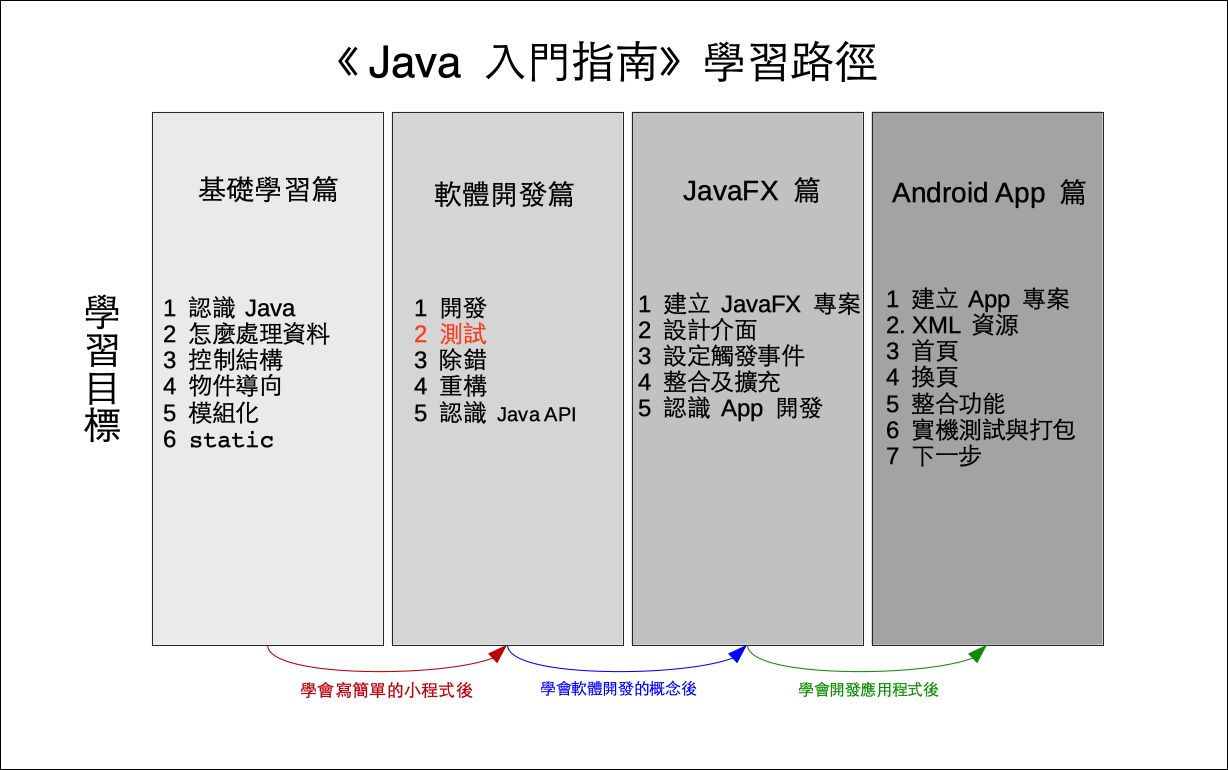# 單元 15 - 繼續測試

～～學習進度表～～Development

Use

Modification

Analysis

Design

Implement

Test

API 中 Math 類別的 random() 方法可產生小於 0 的隨機浮點數，我們將其乘以 10 ，然後強制轉換型態為 int ，便可使 ab 得到 09 的隨機整數。

``````/*
* 檔名：Encrypt03.java
* 作者：張凱慶
* 網站：http://kaiching.org
*/
package encrypt03;

public class Encrypt03 {
// 密碼表字元陣列
private char[] code = new char;

// 建構子
public Encrypt03() {
setCode();
}

// setter
public void setCode() {
int a = 0;
int b = 0;

a = (int) (Math.random() * 10);
b = (int) (Math.random() * 10);

int x, y, m, i;
char c = 'a';
for (i = 0; i < 26; i++) {
x = c;
y = x * a + b;
m = y % 26;
code[i] = (char) (m + 97);
c++;
}
}

// getter
public char[] getCode() {
return code;
}

// 編碼的方法
public String toEncode(String s) {
return s;
}

// 解碼的方法
public String toDecode(String s) {
return s;
}

// 測試的 main()
public static void main(String[] args) {
for (int i = 0; i < 20; i++) {
Encrypt03 e = new Encrypt03();
System.out.println(e.getCode());
}
//String s = "There is no spoon";
//System.out.println(s);
//String s1 = e.toEncode(s);
//System.out.println(s1);
//String s2 = e.toDecode(s1);
//System.out.println(s2);
}
}``````

Math 中的 random()static 方法，因此是直接由 Math 呼叫

``````a = (int) (Math.random() * 10);
b = (int) (Math.random() * 10);``````

``````// 測試的 main()
public static void main(String[] args) {
for (int i = 0; i < 20; i++) {
Encrypt03 e = new Encrypt03();
System.out.println(e.getCode());
}
//String s = "There is no spoon";
//System.out.println(s);
//String s1 = e.toEncode(s);
//System.out.println(s1);
//String s2 = e.toDecode(s1);
//System.out.println(s2);
}``````

``````//String s = "There is no spoon";
//System.out.println(s);
//String s1 = e.toEncode(s);
//System.out.println(s1);
//String s2 = e.toDecode(s1);
//System.out.println(s2);``````

 iloruxadgjmpsvybehknqtwzcf prtvxzbdfhjlnprtvxzbdfhjln qsuwyacegikmoqsuwyacegikmo rajsbktcludmvenwfoxgpyhqzi jqxelszgnubipwdkryfmtahovc mtahovcjqxelszgnubipwdkryf emucksaiqygowemucksaiqygow lszgnubipwdkryfmtahovcjqxe xyzabcdefghijklmnopqrstuvw mtahovcjqxelszgnubipwdkryf iloruxadgjmpsvybehknqtwzcf zhpxfnvdltbjrzhpxfnvdltbjr qzirajsbktcludmvenwfoxgpyh qzirajsbktcludmvenwfoxgpyh iloruxadgjmpsvybehknqtwzcf cgkoswaeimquycgkoswaeimquy cccccccccccccccccccccccccc vxzbdfhjlnprtvxzbdfhjlnprt abcdefghijklmnopqrstuvwxyz hknqtwzcfiloruxadgjmpsvybeanalysis分析
array陣列
class類別
comment註解
design設計
development開發
field屬性
implement建置
method方法
modification修改
software life cycle軟體生命週期
testing測試
use使用

1. The Java™ Tutorials: Declaring Classes
2. The Java™ Tutorials: Declaring Member Variables
3. The Java™ Tutorials: Defining Methods
4. The Java™ Tutorials: Providing Constructors for Your Classes
5. The Java™ Tutorials: Returning a Value from a Method
6. The Java™ Tutorials: Using the this Keyword
7. The Java™ Tutorials: Controlling Access to Members of a Class
8. The Java™ Tutorials: Understanding Class Members
9. The Java™ Tutorials: Initializing Fields
10. The Java™ Tutorials: Beyond Basic Arithmetic

1. 軟體的生命週期分成開發、使用、修改三大階段。
2. 開發階段就是對需求分析，然後設計、建置、測試等等。
3. Java API 中 Mathrandom() 回傳小於 0 的隨機浮點數。
4. 設計、建置完軟體的初始版本就進行測試，如果有問題，就重新檢視設計， Encrypt 的數學公式有問題，因此要研究問題出在哪，再回頭修改程式。

1. 軟體的生命週期分成哪些階段？
2. 設計軟體的時候，該不該重視使用者的體驗經驗？
3. 想一想， Encrypt 的數學公式問題可能發生在哪裡？該如何修正呢？

1. 承接上一個單元的猜數字遊戲，將新專案寫在 Exercise1501 中，把測試答案的部份寫在 Guess 類別的 test() 方法裡，結果回傳布林值。
2. 承上題，建立新專案 Exercise1502 ，在 Guess 類別設計一個 ABCounter() 方法計算 AB 值， AB 值，用屬性記錄，若使用者沒用猜對就印出AB 值。
3. 承上題，加入迴圈直到使用者猜對答案為止，將新專案寫在 Exercise1503 中。